# Exercise 1: Confidence/uncertainty

## Instructions:

This document contains information, questions, R code, and plots.

Hints and reminders are italic

Questions appear in blue.

# R code looks like this.
# Comments are in grey and explain the code

2+2 # add two and two

##  4

# outputs from R appear like the above ^^^^


You should work through the exercise in groups and write answers in a separate document to be submitted on Blackboard by Thursday 31st January.

## A new R function we will use (optimise)

This function provides an easy way for us to optimise over a parameter (find the 'best' value). What we consider the 'best' value of a parameter depends on what criteria we want to optimise. For most of the work we are doing we want to find the parameter that maximises the likelihood. We can use optimise to calculate the likelihood for all possible values of a parameter (within bounds we set) and find the maximum of these.

Things we have to provide to optimise (the arguments optimise takes):

• f, the function to be optimised, for us that is the likelihood.
• lower, the lower bound of parameter values to try.
• upper, the upper bound of parameter values to try.
• maximum, TRUE or FALSE, whether to maximise or minimise the function. We want to maximise.
• Other arugments. We also need to include any other arguments the function in f needs, as well as the one we want to find the optimum of.

## The challenge: management of dragons in Trondheim

You are a team of scientists studying the reintroduction of dragons into Trondheim.

Dragons were reintroduced last year as part of rewilding efforts. The exact species of dragon introduced is a seed eater and so no threat to people or livestock. They also help maintain lower vegetation across the river, which helps kayakers and other river users. Since reintroduction you have been collecting data on these dragons as part of your research. Now the government wants you to present your findings to help them choose a management strategy:

• 1) Business as usual (do nothing)
• 2) Manage the population negatively (shooting)
• 3) Manage the population positively (extra feeding or habitat creation)

From previous work you know that an average population abundance of 35 individuals is needed to maintain the population and keep vegetation under control. An average population abundance of more than 50 individuals will start to put pressure on resources and increase risks of fire.

It is your job to analyse your data and advise the government how to manage the population.

### Analysis one: sex ratio of dragon eggsOne of your team has been collecting data on the sex of dragon eggs. Conveniently male dragons are purple and females are red (even as eggs).

They have collected data from 100 eggs. Their data can be found at (https://www.math.ntnu.no/emner/ST2304/2019v/Week3/sex_ratio_data.csv). This file has a header and is a csv. You can either download and then import it using read.csv() or import directly using the whole we address above.

In the sex column 0 = male and 1 = female.

Remember: to access a particular column of a data frame you use $e.g. sex_ratio_data$Egg_number

First thing we should always do is look at our data. Plot a histogram of the sex column. This is not a pretty graph but lets you see what sort of data you have.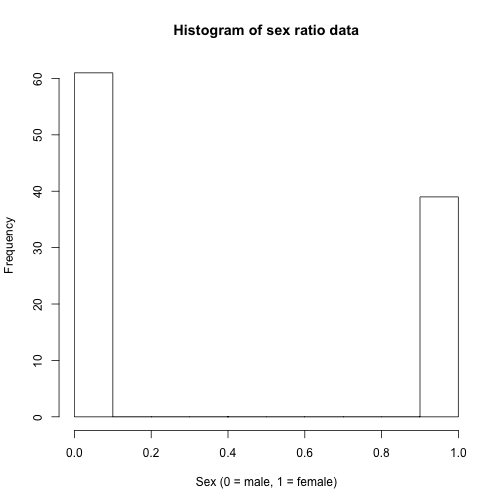From this data you can calculate the observed proportion of female eggs:

1. What is the proportion of female eggs in the observed data? (include one line on how you calculated it)

Now you have the proportion of female eggs that were observed.

But this does not tell us much. You need to complete some statistical analyses to make generalisable statements about the results.

2a. Begin by calculating the likelihood for different probabilities of being female (here being female = getting a success).

• You will need to create an object of your number of successes
• Work out your number of trials (how many times did you try to get a success?)
• Use the dbinom() function to find the likelihoods (hint: use ?dbinom if you can't remember what arguments it takes)
• Try and change the x and y axis labels to something clearer than the defaults (use help and google to find out how)

2b. Then plot the likelihood curve.

3a. At what point along the curve is the maximum likelihood estimate? 3b. How can we find it, mathematically? (hint: you can describe the mathematical process in words it does not have to be the equation)

Below is the code to find the maximum likelihood estimate of the probability of being female in R and plot it on the graph.

This is where we use optimise

# f = the dbinom function we already used above
# We now add an upper and lower bound for the probability (0 and 1)
# We still need to include the arguments of the number of successes
# and number of trials. dbinom needs these to run
# We have set maximum to TRUE
MLE.binom <- optimise(f=dbinom, lower=0, upper=1, x=NSuccess,
size=NTrials, maximum=TRUE)$maximum #$maximum takes only the maximum value from the output
MLE.binom

##  0.3899995

# Now we plot it

# repeat the plot from above
plot(x=Probabilities, y=likelihoods, type="l", xlab="Probability of female",
ylab="Likelihood")

# add a point at the maximum likelihood
# pch=16 and col="red", makes the point red and filled in
points(MLE.binom, max(likelihoods), col="red", pch=16)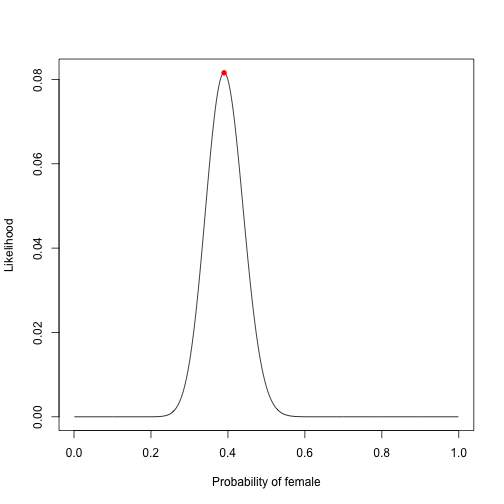You should now have a plot of the likelihoods and the probability with the maximum likelihood. Although you have managed to calculate this, it is not the whole picture.

4a. Why is the maximum likelihood estimate alone not enough to draw statistical conclusions? 4b. What other information should we include?

Below is the code to calculate the confidence intervals for the maximum likelihood estimate and plot them, using a normal approximation.

5.Write a definition for the standard error.

r <- 39 # number of successes
N <- 100 # total number of trials
p <- 0.39 # proportion of success
phat <- r/N
stderr <- sqrt(phat*(1-phat)/N) # creating the standard error

# create normal approximation of likelihood
likelihoods.approx <- dnorm(Probabilities, phat, stderr)
# scale this by the maximum likelihood (becomes relative likelihood)
likelihoods.approx <- likelihoods.approx/max(likelihoods.approx)

# plot the previous likelihood (binomial)
plot(x=Probabilities, y=likelihoods, type="l", ylab="Likelihood", xlab="Probability")
# add a line for the new normal approximation likelihood
# need to multiply the relative likelhoods by the maximum from the binomial
lines(Probabilities, likelihoods.approx*max(likelihoods), col="red")

# calculate the lower and upper confidence intervals using the standard error
CI_lower <- MLE.binom - 1.96*stderr
CI_upper <- MLE.binom + 1.96*stderr
# display the rounded result
round(c(CI_lower, CI_upper), 2)

##  0.29 0.49

# mark the CIs on the plot - can do with lines, polygons or points
# I have chosen lines because it is simple code
abline(v=0.39) # MLE line
abline(v=c(CI_lower, CI_upper), col="red") # CI lines
# add text labels (\n creates a new line)
text(x=0.2, y=0.04, labels="Lower\nCI", col="red")
text(x=0.57, y=0.04, labels="Upper\nCI", col="red")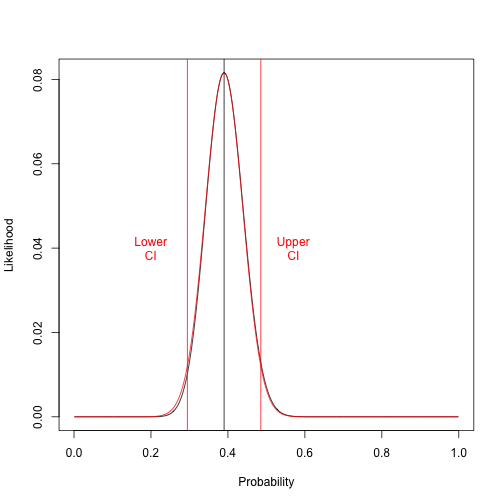6. Do the dragons have a skewed sex ratio as eggs (is it different to 50-50)? Use the confidence intervals in your answer. Explain your answer

### Analysis two: adult dragon numbers

Another member of your team has been collecting data on the number of adult dragons in Trondheim. They have set up a feeding station along the river. They have counted the number of dragons that visited the feeding station in a single day. The number of dragons they counted was 30.

This is just a single count, if you repeated the count on more days each one would differ. Just like the amount of land and sea we sampled differed each time we threw the beach ball.

Here we assume that our count data has come from a Poisson distribution. Count data, can only be whole numbers that are always positive, the Poisson distribution also has these properties. Therefore it is the appropriate choice.

In the same way as for binomial data, we want to find what parameter values (but this time for the Poisson distribution), which produce the highest likelihood given our observed data, those that are most likely to give rise to our data.

Here you only have information on when you did see a dragon, not how many you did not see one (there is no record of failures).

You need to save your number of observations as an object in R.

The Poisson distribution is characterised by a single parameter. Lambda $$\lambda$$ which is the mean (and also the variance) of the distribution. As with the binomial distribution (and p, the probability), we can also find the maximum likelihood estimate for the Poisson, but here we are finding the likelihood of different $$\lambda$$ (means).

Below you can see the code to find the likelihoods of different $$\lambda$$ and then plot them. Details are as follows:

Remember: we can only have whole dragons

• x is the observed count, in the same way observed successes was for the binomial.
• Use the dpois() function to find the likelihoods (dpois(x=ObservedData, lambda=TestLambdas) or use ?dpois)
# Begin by creating a sequence of lambdas to find likelihoods for
lambdas <- seq(1,70,1) # 70 lambdas (means)

# Calculate the likelihoods
likelihoods.Pois <- dpois(x=ObservedAbundance, lambdas)

# plot the outcome
plot(lambdas, likelihoods.Pois, type="l", ylab="Likelihood", xlab="Lambda (mean)")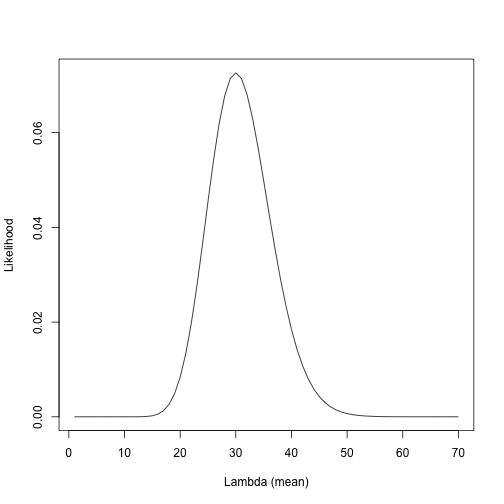To find the maximum likelihood we again use optimise.

# replot the outcome
plot(lambdas, likelihoods.Pois, type="l", ylab="Likelihood", xlab="Lambda (mean)")

# This time we use optimise on the dpois function used above
# Set lower and upper to the bounds of your lambdas above
MLE.Pois <- optimise(dpois, lower=0, upper=70, x = ObservedAbundance,
maximum = TRUE)\$maximum
MLE.Pois

##  30

# plot at point at the maximum likelihood
points(MLE.Pois, max(likelihoods.Pois), col="blue", pch=16)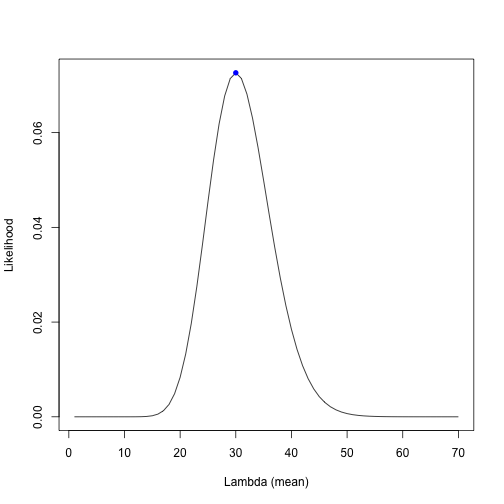You now have a maximum likelihood estimate for the mean abundance ($$\lambda$$) of adult dragons in Trondheim. As with the sex ratio though, this is not all of the information that we need for statistical inference. We would also like a measure of our confidence (uncertainty) in our estimate.

The following code calculates the confidence intervals for the maximum likelihood estimate of $$\lambda$$

Remember: the variance is the square of the standard deviation. dnorm() takes the standard deviation = sqrt(lambda)

# calculate approximate likelihoods from normal distribution
# mean is MLE from Poisson and standard deviation is square root of MLE Poisson
likelihoods.approx.Pois <- dnorm(lambdas, MLE.Pois, sqrt(MLE.Pois))
likelihoods.approx.Pois <- likelihoods.approx.Pois/max(likelihoods.approx.Pois)

plot(lambdas, likelihoods.Pois, type="l", ylab="Likelihood", xlab="Lambda (mean)")
lines(lambdas, likelihoods.approx.Pois*max(likelihoods.Pois), col="blue")

stderr_Pois <- sqrt(MLE.Pois) # standard deviation as normal approximation
CI_lower <- MLE.Pois - 1.96*stderr_Pois
CI_upper <- MLE.Pois + 1.96*stderr_Pois
round(c(CI_lower, CI_upper),2)

##  19.26 40.74

# Exact CIs by knowing the maths
## You do not need to know this!!! It's just here so we can plot them.
ExactCI <- qchisq(p=c(0.025, 0.975), df = 2*MLE.Pois)/2

# Mark on the plot - can do with lines, polygons or points
# This plots a polygon for the exact confidence intervals
InExactCI <- lambdas > ExactCI & lambdas < ExactCI

# This code shades the plot under the curve and between the exact confidence intervals
polygon(x=c(lambdas[InExactCI], max(lambdas[InExactCI]), min(lambdas[InExactCI])),
y=c(likelihoods.Pois[InExactCI], 0,0), border=NA, col="grey70")

# Then mark on the plot approximate intervals
# can do with lines, polygons or points
# This one is a line
abline(v=30) # MLE line
abline(v=c(CI_lower, CI_upper), col="blue") # CI lines
text(x=10, y=0.04, labels="Lower\nCI", col="blue")
text(x=50, y=0.04, labels="Upper\nCI", col="blue")

# Adds a legend (key) to the plot in the top right corner
# Labelled Exact CI and Approx CI, with a grey and blue filled square and no line around the edge (bty = 'n')
legend("topright", c("Exact CI", "Approx CI"),
fill=c("grey70", "blue"), bty='n')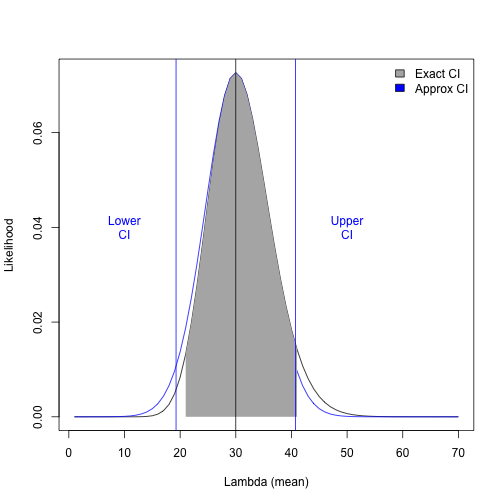The approximate 95% confidence intervals for the Poisson distribution are 19 to 41 (to nearest whole dragon) with a maximum likelihood estimate of 30 individuals.

7. Interpret the confidence intervals here. What do they represent?

8. Are you happy with the normal approximation and the confidence intervals here? Why/Why not?

### Recommendation

Your team have analysed two sets of data on the dragon population in Trondheim. Now you have to create a management recommendation for the local government.

9. Produce a recommendation for the government. It should include:

• Which management option you would recommend based on your analyses.
• Why you suggest this particular option (using analyses to support your reasons).
• Suggest some biological reasons for what you have found (i.e. if the population seems lower/higher than you might expect, why could this be? Does the data you looked at tell you anything about reasons?)
• Ideas for future studies you could conduct to understand more about this population, such as some data you could collect or a question you might want to ask (e.g. do dragons prefer some areas to others?)